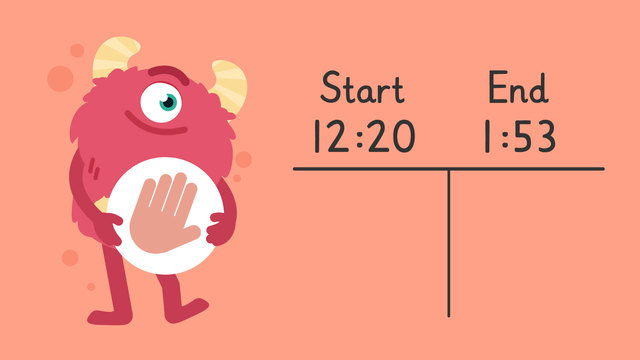# Elapsed Time—Let's Practice!Rating

Be the first to give a rating!
The authorsTeam Digital
Elapsed Time—Let's Practice!
CCSS.MATH.CONTENT.4.MD.A.2

## Elapsed Time—Let's Practice! exercise

Would you like to apply the knowledge you’ve learned? You can review and practice it with the tasks for the video Elapsed Time—Let's Practice!.
• ### How long did it take to walk to the swimming pool?

Hints

How many minutes have been added on each time? Place the correct number of minutes into each box.

Between 1:17 and 1:20 there are 3 minutes. How long between the other times?

Remember, add up the minutes to find the total.

Solution

It took Ajay 48 minutes to walk to the swimming pool.

• There are 3 minutes between 1:17 and 1:20.
• There are 10 minutes between 1:20 and 1:30.
• There are 30 minutes between 1:30 and 2:00.
• There are 5 minutes between 2:00 and 2:05.
• 3 + 10 + 30 + 5 = 48 minutes
• ### Can you find the elapsed time?

Hints

Do we need to add any hours on?

Add the hours first, then look at the minutes.

You could find the elapsed time using these times. How many minutes between 3:35 and 3:40? How many minutes between 3:40 and 3:48?

Solution

The correct elapsed time is 1 hour 13 minutes.

• There is 1 hour between 2:35 and 3:35.
• There are 5 minutes between 3:35 and 3:40.
• There are 8 minutes between 3:40 and 3:48.
• 5 + 8 = 13
• 1 hour + 13 minutes = 1 hour 13 minutes
• ### How long did it take to prepare for the party?

Hints

How much time has passed between the start time and 2:02?

How much time has passed between 2:02 and 2:10?

This is what the first part of the table should look like.

Solution

The time it took Bella to prepare for the party was 2 hours 23 minutes.

• There are 2 hours between 12:02 and 2:02.
• There are 8 minutes between 2:02 and 2:10.
• There are 10 minutes between 2:10 and 2:20.
• There are 5 minutes between 2:20 and 2:25.
• 8 + 10 + 5 = 23 minutes
• 2 hours + 23 minutes = 2 hours 23 minutes
• ### Can you find the elapsed time?

Hints

For the first question, you could start a table like this. How much time has passed from 3:07 to 4:07?

Once you have found the hours, carry on finding the minutes. This one has been continued but make sure you find the minutes until you reach the end time.

Solution

1. The table above shows us that there is 1 hour, 48 minutes between 3:07 and 4:55.
• There is 1 hour between 3:07 and 4:07.
• There are 3 minutes between 4:07 and 4:10.
• There are 20 minutes between 4:10 and 4:30.
• There are 20 minutes between 4:30 and 4:50.
• There are 5 minutes between 4:50 and 4:55.
• 3 + 20 + 20 + 5 = 48 minutes
• 1 hour + 48 minutes = 1 hour 48 minutes

2.12:57 to 4:15: 3 hours, 18 minutes
3.6:30 to 8:36 2 hours, 36 minutes
4.8:47 to 11:53 3 hours, 6 minutes

• ### Can you complete the table to find the elapsed time?

Hints

How many hours have passed from 10:30 - 11:30?

1 hour has passed from 10:30 - 11:30.

Can you now add the hours and minutes to find the total?

To find the minutes, you need to add 10 + 2.

Solution

1 hour 12 minutes has passed from 10:30 - 11:42.

• There is 1 hour between 10:30 and 11:30.
• 10 minutes + 2 minutes = 12 minutes.
• 1 hour + 12 minutes = 1 hour 12 minutes
• ### Mia's Day at the Beach.

Hints

Add on amounts of time to get to friendly numbers. This makes it easier to add.

When finding the time they got home, you need to add 2 hours and 22 minutes onto 5:47, start with the hours and then the minutes. You could break down the minutes to help you.

Solution

On Saturday, Mia and her family went to the beach. They left their house at 8:58 and arrived at the parking lot at 10:52. It took them 1 hour and 54 minutes to get there.

They got out of the car and headed to the beach. Mia wanted to build a sandcastle. She started at 11:03 and finished at 12:31. It took her 1 hour and 28 minutes.

The family enjoyed the beach during the afternoon. They went swimming and played games. They had arrived at the beach at 10:57 and they left at 4:28. They spent 5 hours and 31 minutes at the beach.

They then had dinner before leaving for home at 5:47. It took them 2 hours and 22 minutes to get home. They arrived home at 8:09. What a great day!